## Friday, April 18, 2008

Recently I was working with an organization, building estimation models (rather than classification). They were interested in using linear regression, so I dutifully looked at the distribution,
as shown to the left (all pictures were generated by Clementine, and I also scaled the distribution to protect the data even more, but didn't change the shape of the data).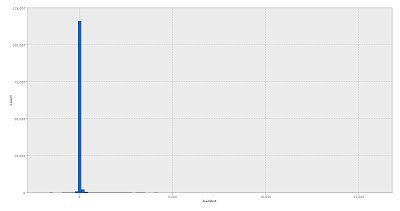There were approximately 120,000 examples. If this were a typical skewed transformation, I would log transform it and be done with it. However, in this distribution there are three interesting problems:

1) skew is 57--heavy positive skew
2) kurtosis is 6180--heavily peaked
3) about 15K of these had value 0, contributing to the kurtosis value

So what to do? One answer is to create the log transform, but maintain sign, using sgn(x)*log10( 1 + abs(x) ). This picture looks like this: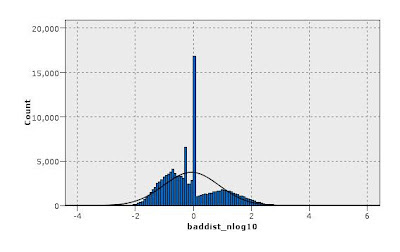This takes care of the summary statistics problems, as skew became 0.6 and kurtosis -0.14. But it doesn't look right--the spike at 0 looks problematic (and turned out that it was). Also, the distribution actually ends up with two ~normal distributions of different variance, one to the left and one to the right of 0.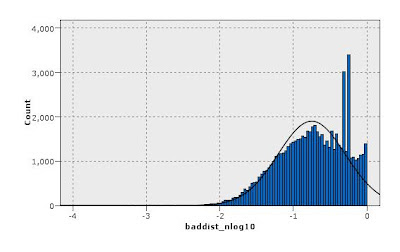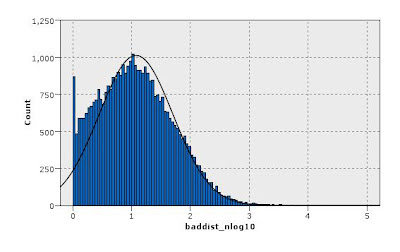Another approach to this is to use the logistic transform 1 / ( 1 + exp(-x/A) ) where A is a scaling factor. Here are the distributions for the original distribution (baddist), the log-transformed version (baddist_nlog10), and the logistic transformed with 3 values of A: 5, 10, and 20, with the corresponding pictures for the three logistic transformed versions.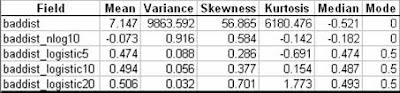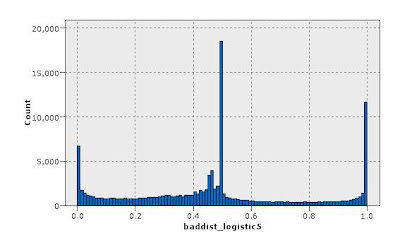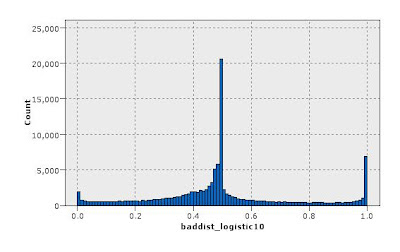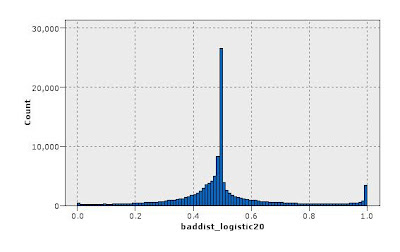Of course, going solely on the basis of the summary statistics, I might have a mild preference for the nlog10 version. As it turned out, the logistic transform produced "better" scores (we measure model accuracy by how well the model rank-ordered the predicted amounts, and I'll leave it at that). That was interesting in of itself since none of the distributions really looked very good. However, another interesting question was which value of "A" to use: 5, 10, 20 (or some other value I don't show here). We found the value that worked best for us, but because of the severity of the logistic transform in how it scales the tails of the distribution, the selection of "A" depended on which range of the target values we were most interested in rank-ordering well. The smaller values of A produced bigger spikes at the extremes, and therefore the model did not rank-order these values well (these models did better on the lower end of distribution magnitudes). If we wanted to identify the tails better, we should increase the scaling factor "A" and it did in fact improve the rank-ordering at the extremes.

So, in the end, the scaling of the target value depends on the business question being answered (no surprises here). So now I open it up to all of you--what would you do? And, if you are interested in this data, I have it on my web site that you can access here.

Will Dwinnell said...

One possibility is to break up the data into three groups: negative values, zeros and positive values and deal with them using separate models.

Pros:
- Sometimes this works well

Cons:
- Not feasible if there are many variables with such awkward distributions
- Does not directly address the overlapped normal sub-populations you have identifiedAnonymous said...

Dean,
Just wondering why you feel the need to transform the data at all before you have actually tried modelling it? It might be that the independent variables can fully explain the distribution, in which case you will have been wasting your time.
Could you not explain to the client that linear regression is not really the best tool. You have to sometimes transform the data to overcome the limitations of the algorithm - why not just use a more flexible algorithm in the first place?

Dean Abbott said...

First, linear regression was required, and of course transforming variables is the typical thing one does so that the output variable distribution is close to normal.

Given that, you're right that one doesn't necessarily need to transform the variable. If one doesn't, what happens is that the squared-error metric regression uses will bias the model toward the larger magnitudes, resulting in better fits at the upper end, but poorer fits of the data in the center of density of the distribution.

Now this may be just fine if your business objective is to predict accurately at the larger values, but if you are rank-ordering the data and will select cases that are in the center of the distribution, the rank-ordering or predicted values may not be very good at all once you get deep enough into the list.

What we found here was that the logistic transform was a nice compromise--we could adjust which range of the target variable was of most interest and scale appropriately.

If I were using a neural net, on the other hand, I probably wouldn't do this (though still might--they are squared-error algorithms after all), but would decide based upon empirical evidence.

Any other thoughts?

By the way, Will, I like that approach as well and have done so in the past. If the models are good at breaking the data into the three groups, this is a very nice solution.Anonymous said...

The data I work with (telco) is often extremely skewed (I reckon most ‘human’ data is)

The method I use to remove outliers with best results isn't a mathematical one, but is fairly quick to process in a data warehouse and also leans itself well to charts and business reports. I band the column/variable into percentiles by count, so each value from 1 to 100 has an equal number of customers (rows) in it.

That way you can easily grab you top 5% or 10% of customers (for example by spend) and if values of the whole population increase (or decrease) over time your predictive model won’t suffer as much.

I use a simple backpropagation neural net with inputs including percentiles.

btw - if you are using Clementine, beware of the binning node, it wasn't very efficent in Clementine version 10 (maybe improved since then). I use sometimes use the QUARTILE function in Teradata.

Cheers

Tim Manns

Dean Abbott said...

Tim--very nice method, and this would take care of the problem here, I'm sure. I've done the same on occasion, though hadn't thought of it here. We actually did use that exact methodology to map the logistic transformed variable back to the natural units.

By the way, when I use binning in Clementine 10 or 11, I usually generate a Derive node anyway, so that I avoid using the binning node altogether in the final stream. I haven't noticed a problem in 11 (Clem 11 also lets you see the cut points on the fly via an apply button, whereas Clem 10 forced you to "run" the node first in the stream).Anonymous said...

Hi,can anyone enlighten me about the the pro's and con's of doing predictive model using data sets that contain "0" value.I'm using daily data where certain day the value of data is "0". My supervisor advise me to do weekly aggregate data but I'm somehow think that will not produce genuine result.

thanks

Pedro said...

Dear Friends,
I have a similar problem with my output target fro training my Neural network... I want to predict not the stock price (I dont believe on that) but in the direction...generating one of 3 possible signals... -1, 0 and 1 (sell, hold and buy)... but the utpur is a normal distribution with 80% of the sinals with 0.. which make the Neural network assume all the values as 0 to get the accuracy at least of 80%...

What you suggest? include more sell and buy signals based for example on the standard deviation and mean?
Best regards,
Pedro

www.pedrocgd.blogspot.comJill Williams said...

Hi guys-

Hope you see and wont mind responding to an old post.

Dean, when you created the regression on the transformed dependent variable, how did you interpret the outcome (the coefficients)? In terms of the transformed dependent (and did this satisfy the client)?

Thanks!!

Dean Abbott said...

Jill:

That is one of the biggest problems I find with transforming variables in this way--interpretation has to be transformed as well. So if a coefficient is 2.0, that indicates that one unit of the input results in a two unit change in the output. If the input units are log units, this isn't a linear relationship with the output anymore but rather a log relationship.Jill Williams said...

Dean, Thanks! So you would describe to the client that a one unit increase in X leads to a a units increase (decrease) in logistic (Y)?